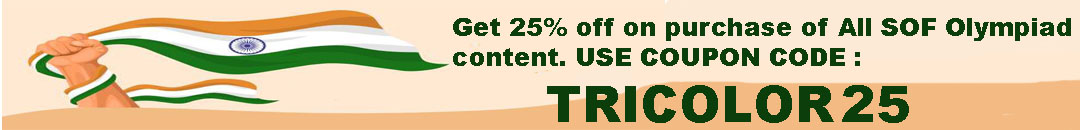# User ForumSubject :IMO    Class : Class 6

## Ans 1:

Class : Class 6
This is so easy that it does not belong in the achiever's section.

Class : Class 6

Class : Class 1

## Ans 4:

Class : Class 6
isn't the second statement place value? then place value will be ten crores

Class : Class 6

## Ans 6:

Class : Class 8

Subject :IMO    Class : Class 7

Class : Class 8

Class : Class 8

Class : Class 9

Class : Class 5

## Ans 5:

Class : Class 8
answer of this question is c.if it is b then rectify it.

Class : Class 9
c-3/2

Class : Class 8

Class : Class 10

## Ans 9:

Class : Class 8

Subject :IMO    Class : Class 8

Class : Class 8

## Ans 2:

Class : Class 9

Subject :IMO    Class : Class 3

## Ans 1:

Class : Class 3
the question is wrong. there are 59 thousands in the answer

Class : Class 4
B

Class : Class 4
B

## Ans 4:

Class : Class 4

Subject :IMO    Class : Class 4

Subject :IMO    Class : Class 4

## Ans 1:

Class : Class 5
The answer is C as the question says that 4 diamonds is 1240 and 1240 divided by 4 is 310 and if we subtract a diamond from 1000 which means 1000-310,the answer comes as one square which is 690 and if we subtract a diamond from a square which means 690-310,the answer comes as 380.

Class : Class 8
380

Class : Class 4
C

Class : Class 4
Option C 380

## Ans 5:

Class : Class 5
Answer is C as the question says that 4 diamonds is 1240,we have to divide to get a diamond's value, which is 1240 divided by 4, the answer comes as 310. If we subtract a diamond from 1000, which is 1000-310, the answer comes as a square, which is 690. If we subtract a diamond from a

Subject :IMO    Class : Class 3

## Ans 1:

Class : Class 4
No!

Subject :IMO    Class : Class 5

Class : Class 7
(B) 2.4 kg

## Ans 2:

Class : Class 6

Subject :IMO    Class : Class 3

Class : Class 6
60 min